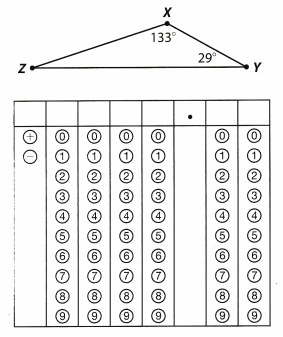Refer to our Texas Go Math Grade 6 Answer Key Pdf to score good marks in the exams. Test yourself by practicing the problems from Texas Go Math Grade 6 Module 15 Quiz Answer Key.

15.1 Determining When Three Lengths Form o Triangle

Determine whether the three side lengths form a triangle.

Question 1.
3, 5, 7 ____
Find the sum of the two sides of the triangle then compare to the third side.
3 + 5 :> 7 add the two sides and compare to the third side
8 > 7 the sum of the two sides is greater than the third side

3 + 7;’ 5 add the two sides and compare to the third side
10 > 5 the sum of the two sides is greater than the third side

5 + 7> 3 add the two sides and compare to the third side
12 > 3 the sum of the two sides is greater than the third side

Yes, the given side lengths can form a triangle.

Question 2.
9, 15, 4 ____
Find the sum of the two sides of the triangle then compare to the third side.
9 + 15 > 4 add the two sides and compare to the third side
24 > 4 the sum of the two sides is greater than the third side

15 + 4 > 9 add the two sides and compare to the third side
19 > 9 the sum of the two sides is greater than the third side

9 + 4> 15 add the two sides and compare to the third side
13 ≯ 15 the sum of the two sides is less than the third side

The given side lengths cannot be used to form a triangle because two sides will have a sum of less than the third side.

Question 3.
17, 5, 23 _________________
Find the sum of the two sides of the triangle then compare to the third side.
17 + 5 > 23 add the two sides and compare to the third side
22 ≯ 23 the sum of the two sides is less than the third side

5 + 23> 17 add the two sides and compare to the third side
28 > 17 the sum of the two sides is greater than the third side

17 + 23 > 5 add the two sides and compare to the third side
40 > 5 the sum of the two sides is less than the third side

The given side lengths cannot be used to form a triangle because two sides will have a sum of less than the third side.

Question 4.
28, 16, 38 _________________
Find the sum of the two sides of the triangle then compare to the third side.
28 + 16 > 38 add the two sides and compare to the third side
44 > 38 the sum of the two sides is greater than the third side

28 + 38 > 16 add the two sides and compare to the third side
66> 16 the sum of the two sides is greater than the third side

16 + 38 > 28 add the two sides and compare to the third side
54 > 28 the sum of the two sides is greater than the third side

The given side lengths can be used to form a triangle.

15.2 Sum of Angle Measures in a Triangle

Find the unknown angle measures.

Question 5.
_________________Determine the unknown angle by adding the given angles and subtracting the result from 180°.
41° + 112° + x = 180° substitute for the sum of angle measures in triangle
153° + x = 180° sum of the two angles
153° + x – 153° = 180° – 153° subtract 153° from both sides of the equation
x = 27° measure of the unknown angle

The unknown angle measure 27°.

Question 6.
_________________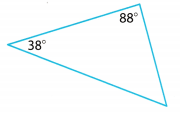Determine the unknown angle by adding the given angles and subtracting the result from 180°.

38° + 88° + x = 180° substitute for the sum of angle measures in triangle
126° + x = 180° sum of the two angles
126° + x – 126°= 180° – 126° subtract 126° from both sides of the equation
x = 54° measure of the unknown angle

The unknown angle measures 54°.

15.3 Relationships Between Sides and Angles in a Triangle

Match each of the given measures to the correct side or angle.

Question 7.
11, 7.5, 13
_________________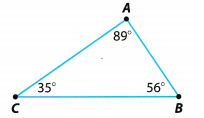Determine the side lengths of the triangle.
AB = 7.5 opposite the smallest angle
AC = 11 opposite the midsize angle
BC = 13 opposite the largest angle

Correct sides of the triangle according to angle measurement:
a. shortest side – segment AB
b. midsize side – segment AC
c. longest side – segment BC

Question 8.
24°, 44°, 112°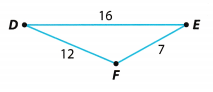Determine the angles based on the given side lengths of the triangle.
∠D = 24° opposite the shortest segment
∠E = 44° opposite the midsize segment
∠F = 112° opposite the longest segment

Correct angle according to the side lengths of the triangle
a. ∠D – smallest angle
b. ∠E-mid size angle
c. ∠F – largest angle

Essential Question

Question 9.
How can you describe the relationships among angles and sides in a triangle?
The angles and sides are related in terms of their opposites. In a triangle, the measure of the angles or sides will determine the size of the angle or side opposite to it. The larger angle is opposite the longest side, the midsize angle is opposite the midsize side, and the smaller angle is opposite the shorter side.

The side lengths in a triangle indicates the size of angles opposite to them.

Texas Go Math Grade 6 Module 15 Mixed Review Texas Test Prep Answer Key

Selected Response

Question 1.
The two longer sides of a triangle measure 16 and 22. Which of the following is a possible length of the shortest side?
(A) 4
(B) 6
(C) 11
(D) 19
(C) 11

Explanation:

a. 16, 22, 4
Find the sum of the two sides of the triangle then compare to the third side.
16 + 22 > 4 add the two sides and compare to the third side
38 > 4 the sum of the two sides is greater than the third side

22 + 4> 16 add the two sides and compare to the third side
26 > 16 the sum of the two sides is greater than the third side

4 + 16> 22 add the two sides and compare to the third side
20 ≯ 22 the sum of the two sides is less than the third side

b. 16, 22, 6
Find the sum of the two sides of the triangle then compare to the third side.

16 + 22 > 6 add the two sides and compare to the third side
38 > 6 the sum of the two sides is greater than the third side

22 + 6> 16 add the two sides and compare to the third side
28 > 16 the sum of the two sides is greater than the third side

6 + 16 > 22 add the two sides and compare to the third side
22 ≯ 22 the sum of the two sides is not greater than the third side

c. 16, 22, 11
Find the sum of the two sides of the triangle then compare to the third side.

16 + 22 > 11 add the two sides and compare to the third side
38 > 11 the sum of the two sides is greater than the third side

22 + 11 > 16 add the two sides and compare to the third side
33 > 16 the sum of the two sides is greater than the third side

11 + 16 > 22 add the two sides and compare to the third side
27 > 22 the sum of the two sides is greater than the third side

d. 16, 22, 19
Find the sum of the two sides of the triangle then compare to the third side.
16 + 22 > 19 add the two sides and compare to the third side
38 > 19 the sum of the two sides is greater than the third side

22 + 19> 16 add the two sides and compare to the third side
41 > 16 the sum of the two sides is greater than the third side

19 + 16 > 22 add the two sides and compare to the third side
35 > 22 the sum of the two sides is greater than the third side

The shortest side of the triangle is C. 11

Question 2.
Part of a large metal sculpture will be a triangle formed by welding three bars together. The artist has four bars that measure 12 feet, 7 feet, 5 feet, and 3 feet. Which bar could not be used with two of the others to form a triangle?
(A) the 3-foot bar
(B) the 5-foot bar
(C) the 7-foot bar
(D) the 12-foot bar
(D) the 12-foot bar

Explanation:
Find the sum of the two sides of the triangle then compare to the third side.
7 + 5 > 3 add the two sides and compare to the third side
12 > 3 the sum of the two sides is greater than the third side

7 + 3 > 5 add the two sides and compare to the third side
10 > 5 the sum of the two sides is greater than the third side

3 + 5 > 7 add the two sides and compare to the third side
8 > 7 the sum of the two sides is greater than the third side

The D. 12-foot bar cannot be used in combination with other two measures to form a triangle.

Question 3.
What is the measure of the missing angle in the triangle below?(A) 39°
(B) 49°
(C) 59°
(D) 69°
(C) 59°

Explanation:
Determine the unknown angle by adding the given angles and subtracting the result from 180°.
65° + 56° + x = 180° substitute for the sum of angle measures in triangle
121° + x = 180° sum of the two angles
121° + x – 121° = 180° – 121° subtract 121° from both sides of the equation
x = 59° measure of the unknown angle

The measure of the missing angle is C. 59°.

Question 4.
The measure of ∠A in ∆ABC is 88°. The measure of ∠B is 60% of the measure of ∠A. What is the measure of ∠C?
(A) 39.2°
(B) 52.8°
(D) 91°
(D) 127.2°
(A) 39.2°

Explanation:
Determine the size of the unknown angle.
m∠B = 0.60 × 88° multiply the measure of ∠A by 60%
= 52.8° measure of ∠B
m∠A + m∠B + m∠C = 180°
88° + 52.8° + x = 180° substitute for the sum of angle measures in triangle
140.8 + x = 180° sum of two angles
140.8° + x – 140.8° = 180 – 140.8° subtract 140.8° from both of the equation
x = 39.2° measure of the unknown angle
The measure of ∠C is A. 39.2°.

Question 5.
Which of these could be the value of x in the triangle below?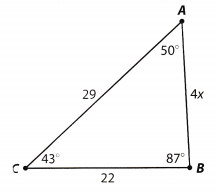(A) 5
(B) 6
(C) 7
(D) 10
(A) 5

Explanation:
Substitute the value for x
a. 4 × 5 = 20 multiply the given number by 5
side lengths of 29 – 22 – 20

b. 4 × 6 = 24 multipLy the given number by 6
side lengths of 29 – 22 – 24

c. 4 × 7 = 28 multiply the given number by 7
side lengths of 29 – 22 – 28

d. 4 × 10 = 40 multiply the given number by 10
side lengths of 29 – 22 – 40

The unknown side length of the triangle is the opposite side of the smallest angle which is ∠C. Therefore, the side length is also the shortest side among all the given values which makes the value of x at 5.

The value of x in the triangle is A. 5

Gridded Response

Question 6.
Find m∠Z.